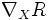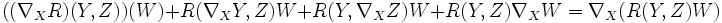# Difference between revisions of "Second Bianchi identity"

## Statement

Let$\nabla$ be a linear connection and$R$ be the Riemann curvature tensor of$\nabla$. Then$R$ can itself be differentiated via$\nabla$, since$R$ is a$(1,3)$-tensor and we can define the connection on all$(p,q)$-tensors. With this meaning, the following cyclic summation is zero:$(\nabla_X R)(Y,Z) + (\nabla_Y R) (Z,X) + (\nabla_Z R)(X,Y) = 0$

This result is known as the second Bianchi identity and also as the differential Bianch identity.

## Proof

To prove this we look more closely at what$\nabla_X R$ means.$\nabla_X R$ must satisfy the following compatibility condition:$((\nabla_X R) (Y,Z)) (W) + R (\nabla_XY,Z) W + R(Y,\nabla_XZ) W + R(Y,Z) \nabla_X W = \nabla_X (R(Y,Z)W)$

We now concentrate on all the other terms.

## Applications

The second Bianchi identity is typically used for the studyo of manifolds of dimension three or higher, since we can choose$X,Y,Z$ as linearly independent at a particular point. Some examples of its application:

• Schur's theorem which states that if the sectional curvature of a manifold of dimension at least 3 depends only on the point and not on the choice of tangent plane, then it is in fact constant at all points. The idea here is to show that the scalar function defined by the sectional curvature has zero derivative. To prove this, we employ the second Bianchi identity by choosing$X,Y,Z$ as linearly independent
• Gradient estimates for scalar curvature, which are used for studying things like the Ricci flow on higher-dimensional manifolds